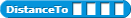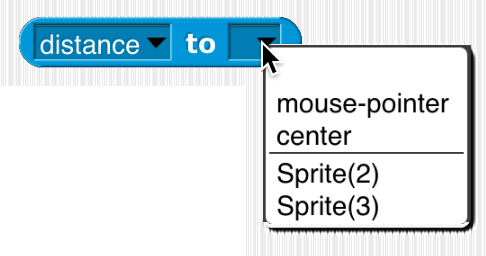# [Feature Request] Create Distance Reporter Block

How the Feature would work: What the block would do is use the Pythagorean Theorem to calculate the distance between two objects. It would take 4 inputs and return one input which would be the distance of two objects.This is what the block would look like. The way I set it up is where the inputs are as follows, "ObjectX1,ObjectX2, ObjectY1, ObjectY2" (Where Each Input is the coordinate of Two Objects). As by the Picture It would be best to have it be a sensing block as what its used for.

Hi! Welcome to the forum.

It's easy enough to build your block in Snap! so it doesn't really have to be a primitive.

But in any case that's not the way we think about things like distances. What you really want isn't the distance between two sets of x,y coordinates; you want the distance between two objects. That's why we let one object ask for its distance from another object:If you really want to know its distance from an x,y coordinate point, you can do that too: